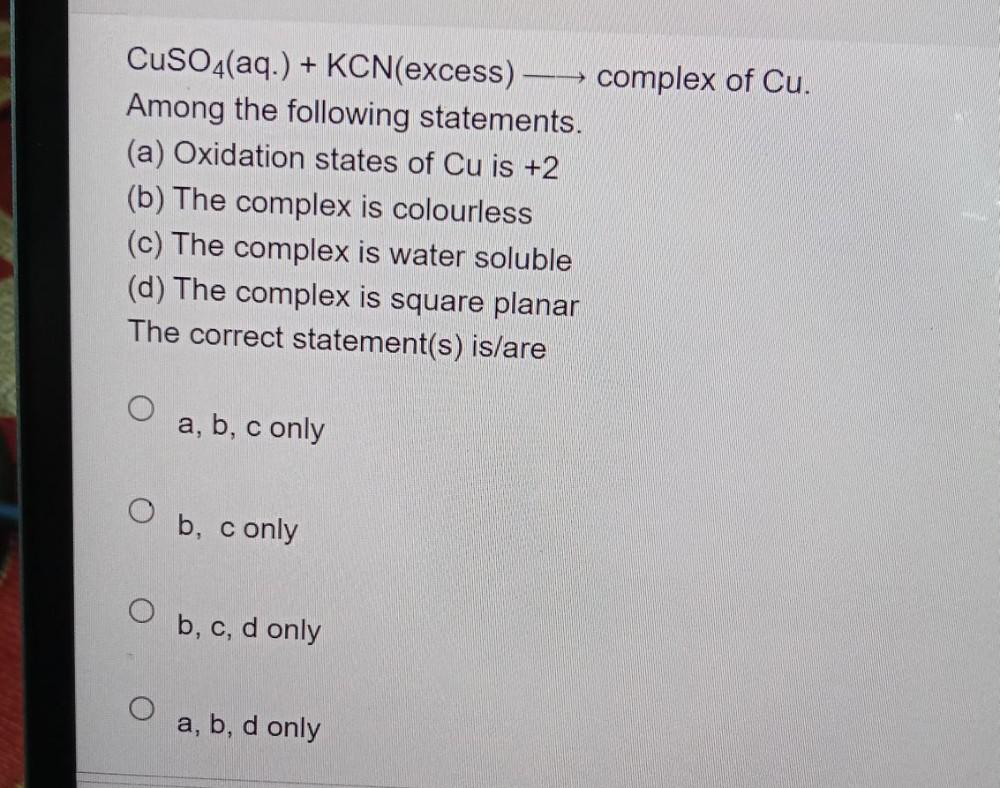Question:

# CuSO4(aq.) + KCN(excess)– complex of Cu. Among the following statements. (a) Oxidation states of Cu is +2 (b) The complex is colCuSO4(aq.) + KCN(excess)– complex of Cu. Among the following statements. (a) Oxidation states of Cu is +2 (b) The complex is colourless (c) The complex is water soluble (d) The complex is square planar The correct statement(s) is/are a, b, c only O b, c only b, c, d only a, b, d only